# PSAT Math : Integers

## Example Questions

### Example Question #1581 : Sat Mathematics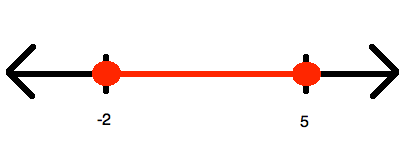Which of the following inequalities is represented by the number line shown above?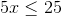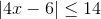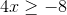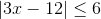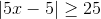Explanation:

Since the inequality represents one range of values between two end points (both of which are included, given the sign being "less than or equal"), you know that whatever you answer, it must be convertible to the form: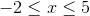Now, you know that it is impossible to get this out of the choices that have no absolute values involved in them. Therefore, the only options that make sense are the two having absolute values; however, here you should choose only the ones that have a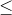, for only that will yield a range like this. Thus, we can try both of our options.

The wrong answer is simplified in this manner: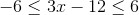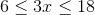And you can stop right here, for you know you will never havefor the left terminus.

The other option is simplified in this manner: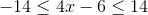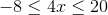This is just what you need!

### Example Question #1 : How To Graph An Inequality With A Number Line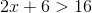Which of the following is a graph for the values ofdefined by the inequality stated above?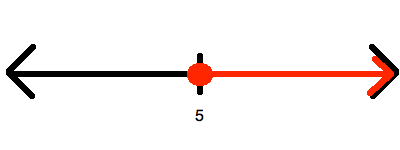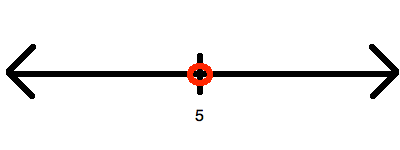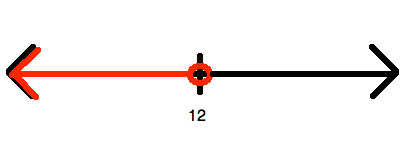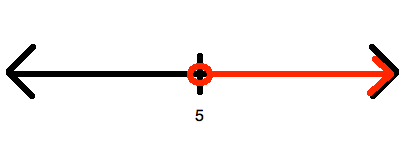Explanation:

Begin by solving for: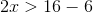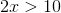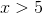Now, this is represented by drawing an open circle at 6 and graphing upward to infinity:### Example Question #1 : Other Number Line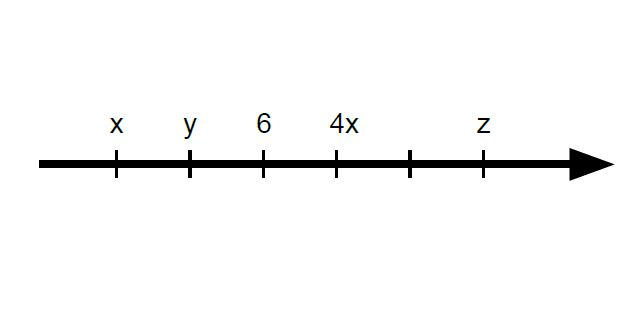If the tick marks are equally spaced on the number line above, what is the average (arithmetic mean) of x, y, and z?

6

8

7

4

5

6

Explanation:

First, we must find out by how much they are spaced by. It cannot be 1, since 4(4) = 16, which is too great of a step in the positive direction and exceeds the equal-spacing limit. 2 works perfectly, however, as 4(2) equals 8 and fits in line with the equal spacing.

Next, we can find the values of x and y since we are given a value of 6 for the third tick mark. As such, x (6 – 4) and y (6 – 2) are 2 and 4, respectively.

Finally, z is 4 steps away from y, and since each step has a value of 2, 2(4) = 8, plus the value that y is already at, 8 + 4 = 12 (or can simply count).

Finding the average of all 3 values, we get (2 + 4 + 12)/3 = 18/3 = 6.

### Example Question #1584 : Sat Mathematics

How many numbers 1 to 250 inclusive are cubes of integers?Explanation:

The cubes of integers from 1 to 250 are 1, 8, 27,64,125,216.

### Example Question #1 : How To Find Value With A Number Line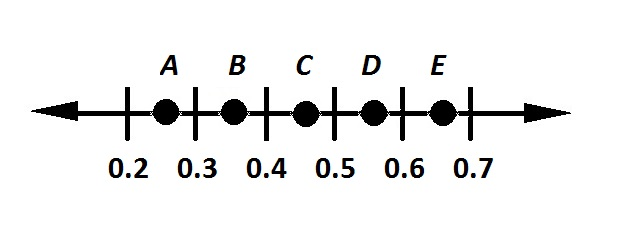Refer to the above number line. Which of the points is most likely the location of the number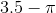?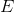Explanation: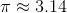, so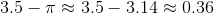Therefore,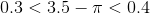On the number line,appears between 0.3 and 0.4 and is the correct choice.

### Example Question #1 : How To Add Negative Numbers

How many elements of the set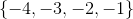are less than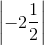?

Four

One

Three

None

Two

Four

Explanation:

The absolute value of a negative number can be calculated by simply removing the negative symbol. Therefore,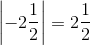All four (negative) numbers in the setare less than this positive number.

### Example Question #13 : Integers

a, b, c are integers.

abc < 0

ab > 0

bc > 0

Which of the following must be true?

b > 0

ac < 0

a + b < 0

a – b > 0

a > 0

a + b < 0

Explanation:

Let's reductively consider what this data tells us.

Consider each group (a,b,c) as a group of signs.

From abc < 0, we know that the following are possible:

(–, +, +), (+, –, +), (+, +, –), (–, –, –)

From ab > 0, we know that we must eliminate (–, +, +) and (+, –, +)

From bc > 0, we know that we must eliminate (+, +, –)

Therefore, any of our answers must hold for (–, –, –)

This eliminates immediately a > 0, b > 0

Likewise, it eliminates a – b > 0 because we do not know the relative sizes of a and b. This could therefore be positive or negative.

Finally, ac is a product of negatives and is therefore positive. Hence ac < 0 does not hold.

We are left with a + b < 0, which is true, for two negatives added must be negative.

### Example Question #61 : Integers

What is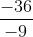?

45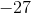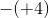Explanation:

A negative number divided by a negative number always results in a positive number.divided byequals. Since the answer is positive, the answer cannot beor any other negative number.

### Example Question #1 : Negative Numbers

Solve for: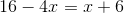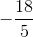Explanation:

Subtractfrom both sides: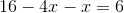, or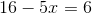Next, subtractfrom both sides: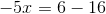, or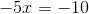Then, divide both sides by: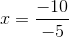Recall that division of a negative by a negative gives you a positive, therefore: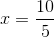or### Example Question #3 : Negative Numbers

If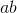is a positive number, and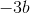is also a positive number, what is a possible value for?Explanation:

Because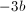is positive,must be negative since the product of two negative numbers is positive.

Because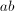is also positive,must also be negative in order to produce a prositive product.

To check you answer, you can try plugging in any negative number for.

### All PSAT Math Resources